Test: Extended Transition Function

# Test: Extended Transition Function - Computer Science Engineering (CSE)

Test Description

## 10 Questions MCQ Test Theory of Computation - Test: Extended Transition Function

Test: Extended Transition Function for Computer Science Engineering (CSE) 2023 is part of Theory of Computation preparation. The Test: Extended Transition Function questions and answers have been prepared according to the Computer Science Engineering (CSE) exam syllabus.The Test: Extended Transition Function MCQs are made for Computer Science Engineering (CSE) 2023 Exam. Find important definitions, questions, notes, meanings, examples, exercises, MCQs and online tests for Test: Extended Transition Function below.
Solutions of Test: Extended Transition Function questions in English are available as part of our Theory of Computation for Computer Science Engineering (CSE) & Test: Extended Transition Function solutions in Hindi for Theory of Computation course. Download more important topics, notes, lectures and mock test series for Computer Science Engineering (CSE) Exam by signing up for free. Attempt Test: Extended Transition Function | 10 questions in 10 minutes | Mock test for Computer Science Engineering (CSE) preparation | Free important questions MCQ to study Theory of Computation for Computer Science Engineering (CSE) Exam | Download free PDF with solutions
 1 Crore+ students have signed up on EduRev. Have you?
Test: Extended Transition Function - Question 1

### The number of tuples in an extended Non Deterministic Finite Automaton:

Detailed Solution for Test: Extended Transition Function - Question 1

For NFA or extended transition function on NFA, the tuple elements remains same i.e. 5.

Test: Extended Transition Function - Question 2

### Choose the correct option for the given statement:Statement: The DFA shown represents all strings which has 1 at second last position   .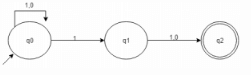Detailed Solution for Test: Extended Transition Function - Question 2

The given figure is an NFA. The statement contradicts itself.

Test: Extended Transition Function - Question 3

### What is wrong in the given definition?Def: ({q0, q1, q2}, {0,1}, δ, q3, {q3})

Detailed Solution for Test: Extended Transition Function - Question 3

q3 does not belong to Q where Q= set of finite states.

Test: Extended Transition Function - Question 4

If δ is the transition function for a given NFA, then we define the δ’ for the DFA accepting the same language would be:Note: S is a subset of Q and a is a symbol.

Detailed Solution for Test: Extended Transition Function - Question 4

According to subset construction, equation 1 holds true.

Test: Extended Transition Function - Question 5

What is the relation between DFA and NFA on the basis of computational power?

Detailed Solution for Test: Extended Transition Function - Question 5

DFA is said to be a specific case of NFA and for every NFA that exists for a given language, an equivalent DFA also exists.

Test: Extended Transition Function - Question 6

If a string S is accepted by a finite state automaton, S=s1s2s3……sn where siϵ∑ and there exists a sequence of states r0, r1, r2…… rn such that δ(r(i), si+1) =ri+1 for each 0, 1, …n-1, then r(n) is:

Detailed Solution for Test: Extended Transition Function - Question 6

r(n) is the final state and accepts the string S after the string being traversed through r(i) other states where I ϵ 01,2…(n-2).

Test: Extended Transition Function - Question 7

According to the given table, compute the number of transitions with 1 as its symbol but not 0: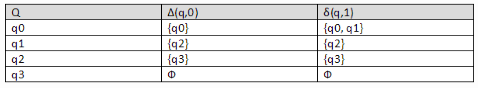Detailed Solution for Test: Extended Transition Function - Question 7

The transition graph is made and thus the answer can be found.

Test: Extended Transition Function - Question 8

From the given table, δ*(q0, 011) =?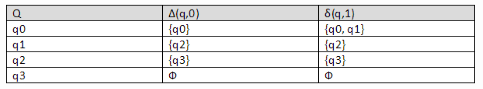Detailed Solution for Test: Extended Transition Function - Question 8

δ*(q0,011) = Uδ*(q0,01) δ (r, 1) = {q0, q1, q2}.

Test: Extended Transition Function - Question 9

Number of times the state q3 or q2 is being a part of extended 6 transition state is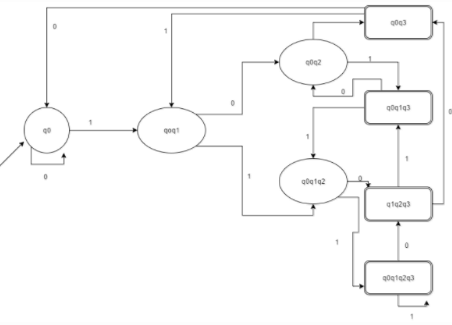Detailed Solution for Test: Extended Transition Function - Question 9

According to the question, presence of q2 or q1 would count so it does and the answer according to the diagram is 6.

Test: Extended Transition Function - Question 10

Predict the missing procedure: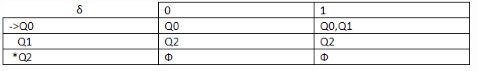1.Δ(Q0, ε) ={Q0},
2.Δ(Q0, 01) = {Q0, Q1}
3.δ(Q0, 010) =?

Detailed Solution for Test: Extended Transition Function - Question 10

According to given table and extended transition state implementation, we can find the state at which it rests.

## Theory of Computation

18 videos|56 docs|44 tests
Information about Test: Extended Transition Function Page
In this test you can find the Exam questions for Test: Extended Transition Function solved & explained in the simplest way possible. Besides giving Questions and answers for Test: Extended Transition Function, EduRev gives you an ample number of Online tests for practice

## Theory of Computation

18 videos|56 docs|44 tests